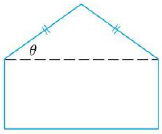Chapter 14, Problem 65RE

Chapter
Section
Textbook Problem

A pentagon is formed by placing an isosceles triangle on a rectangle, as shown in the figure. II the pentagon has fixed perimeter P, find the lengths of the sides of the pentagon that maximize the area of the pentagon.To determine

To find: The lengths of the sides of a pentagon which maximize the area of a pentagon.

Explanation

Given:

Notice that the given pentagon has one rectangle and one isosceles triangle.

Let a and c be the side lengths of the isosceles triangle and the angle between a and c is θ . Let, b and c be the length, breadth of a rectangle.

Thus, the perimeter of the pentagon is fixed.

The above information mentioned in the following Figure 1.

Result used:

“The Lagrange multipliers defined as f(x,y,z)=λg(x,y,z) . This equation can be expressed as fx=λgx , fy=λgy , fz=λgz and g(x,y,z)=k ”.

Calculation:

The area of the triangle is, 12casinθ and the area of a rectangle is bc.

Thus, the area of the pentagon is, f(a,b,c)=12casinθ+bc .

The perimeter of the pentagon is, P=g(a,b,c)=2a+2b+c .

To obtain the value of sinθ in terms of a, b, c, use Pythagoras theorem,

From the triangle ABD, AC=asinθ .

From triangle ABD,

Simplify further as follows.

sinθ=4a2c24a2=12a4a2c2

Substitute sinθ=12a4a2c2 in f(a,b,c)=12casinθ+bc ,

f(a,b,c)=12ca(12a4a2c2)+bc=14c4a2c2+bc

By above result f(a,b,c)=λg(a,b,c) is computed as follows.

f(a,b,c)=λg(a,b,c)fa,fb,fc=λga,gb,gcfa(14c4a2c2+bc),fb(14c4a2c2+bc),fc(14c4a2c2+bc)=λga(2a+2b+c),gb(2a+2b+c),gc(2a+2b+c)ac4a2c2,c,(144a2c2c244a2c2+b)=λ2,2,1

Thus, the value of f(a,b,c)=λg(a,b,c) is, ac4a2c2,c,(144a2c2c244a2c2+b)=λ2,2,1

Still sussing out bartleby?

Check out a sample textbook solution.

See a sample solution

The Solution to Your Study Problems

Bartleby provides explanations to thousands of textbook problems written by our experts, many with advanced degrees!

Get Started

Define sampling with replacement and explain why is it used?

Statistics for The Behavioral Sciences (MindTap Course List)

19. If and , find the following and simplify. (a) (b)

Mathematical Applications for the Management, Life, and Social Sciences

limx5x225x29x+20= a) 1 b) 10 c) d) does not exist

Study Guide for Stewart's Single Variable Calculus: Early Transcendentals, 8th

Give the following tree diagram for an activity, what are the possible outcomes?

Finite Mathematics for the Managerial, Life, and Social Sciences

Given that mAB=106 and mDC=32, find: a m1_ b m2_

Elementary Geometry for College Students